Montclair-The Aplline

# Cases in Cost Management

## Quiz 20 :Montclair-The ApllineStudy FlashcardsLooking for Management Homework Help?## Quiz 20 :Montclair-The ApllinePrepare the five-year financial forecast for this project.
Free
Essay

1. Five year financial forecast for the project is given below: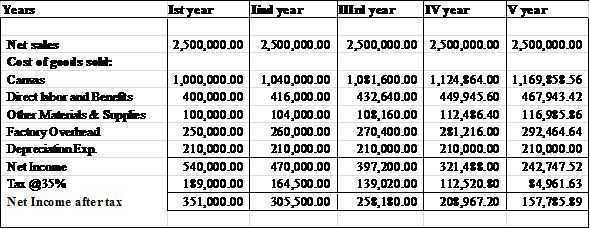Notes:
• Sales is calculated as follows:• All cash cost would probably grow 4% each year from inflation.
• Tax is calculated as 35% on profit.Calculate the IRR, NPV, and Payback.
Free
Essay

Calculation of IRR, NPV, and Payback:
(i)
Initial investment in the project made $1,300,000. NPV is calculated as follows :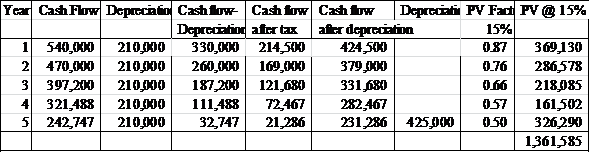NPV calculated is$61,585 which is positive. This means that the project is profitable.
(ii)
IRR stands for internal rate of return.
Internal rate of return (IRR) is that the rate of return at which the NPV from the above investments will become zero. It is used to compare one investment to another.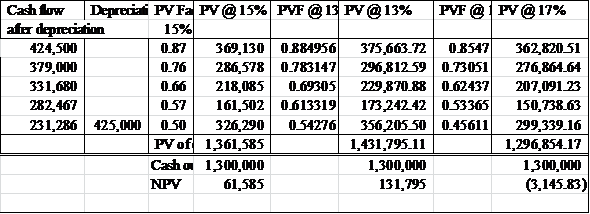For IRR calculation:
Assume: IRR is 13%
NPV at 13% is calculated $131,795. Assume: IRR is 17% NPV at 17% calculated is ($3,145.83)
IRR is calculated as follows:Hence, IRR for the project is 16.91%.
(iii)
Payback for the project is calculated as follows: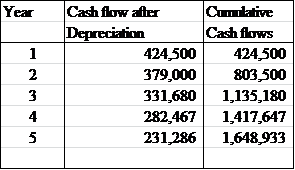Payback period is calculated as follows :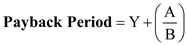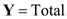number of years before the payback year in this case Y is 3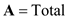remaining to be paid in order to make cumulative cash flow 0 in this case A is $282,467.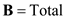Payback amount in entire payback year in this case the amount is$331,680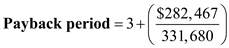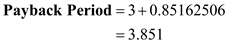Hence, the payback period is 3.851.Is the proposal a good deal for the Navy
Free
Essay